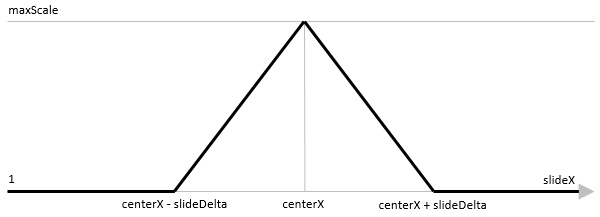Hidden Advanced Features of Ionic's Slides

October 6th 2017

Slides is a very flexible Ionic component for presenting multiple slides to the user who can swipe between them. However, not all its customization options are exposed as Angular Inputs and Outputs or even fully documented. To see all supported options, one can peek into the source code. The only way to learn more about them is to check the Swiper API reference, which the Slides component is based on.

Using Advanced Customization Options

Taking advantage of all these options might still be a challenge, though. As documented, simple properties can be directly accessed:

import { ViewChild } from '@angular/core';
import { Slides } from 'ionic-angular';

class MyPage {
@ViewChild(Slides) slides: Slides;

onSlideTapped() {
console.log(`Slide tapped: \${this.slides.clickedIndex}`);
}
}

Hidden EventEmmiters are a different story. They must be subscribed to from code:

import { ViewChild, OnInit } from '@angular/core';
import { Slides } from 'ionic-angular';

class MyPage implements OnInit {
@ViewChild(Slides) slides: Slides;

ngOnInit(): void {
this.slides.ionSlideProgress.subscribe(progress => this.onProgress(progress));
}
}

All of the hidden properties are only available to interact with after the component is initialized, i.e. in ngOnInit or later.

A Working Example

You might ask, why you'd want to use these hidden features at all. Let's look at a use case that would not be possible without them:In essence, it's a centered Slides component with 5 slides on screen:

<ion-slides slidesPerView="5" centeredSlides="true">
<ion-slide>1</ion-slide>
<ion-slide>2</ion-slide>
<ion-slide>3</ion-slide>
<ion-slide>4</ion-slide>
<ion-slide>5</ion-slide>
<ion-slide>6</ion-slide>
<ion-slide>7</ion-slide>
<ion-slide>8</ion-slide>
<ion-slide>9</ion-slide>
</ion-slides>

But there's a nice zoom effect added to the focused slide - in the center, the slides are scaled up according to the following function:To implement this functionality I take advantage of ionSlideProgress EventEmmiter, which emits a value between 0 and 1 indicating the position between the first and the last slide, whenever the slide position changes:

import { Component, OnInit, ViewChild } from '@angular/core';
import { NavController, Slides } from 'ionic-angular';

class MyPage implements OnInit {
@ViewChild(Slides) slides: Slides;

ngOnInit(): void {
this.slides.ionSlideProgress.subscribe(progress => this.onProgress(progress));
}

onProgress(centerX: number): void {
let maxScale = 2;
let slideCount = this.slides._slides.length;
let slideDelta = 1 / (slideCount - 1)
let slope = (maxScale - 1) / slideDelta;
for (let slideIndex = 0; slideIndex < slideCount; slideIndex++) {
let slideX = slideIndex * slideDelta;
let slideScale = 1;
if (slideX > centerX - slideDelta) {
if (slideX <= centerX) {
slideScale += (slideX - (centerX - slideDelta)) * slope;
} else if (slideX < centerX + slideDelta) {
slideScale += ((centerX + slideDelta) - slideX) * slope;
}
}
this.slides._slides[slideIndex].style.transform = `scale(\${slideScale})`;
}
}
}

Most of the onProgress method is calculating the scale function value for each slide based on its distance from the current center value. I also use the _slides property to access individual slides and set their scale transform.

Although the calculation is correct, there is still a visible glitch in scaling when I end the swipe as the slides transition into their snapped positions:The glitch is caused by my function immediately setting the final value when the swipe ends while the position is still in transition. To fix the issue, I must also apply the transition to the scale transformation when the swipe ends and disable it again when the swipe starts:

ngOnInit(): void {
this.slides.ionSlideProgress.subscribe(progress => this.onProgress(progress));
this.slides.ionSlideTouchStart.subscribe(() => this.toggleTransitions(false));
this.slides.ionSlideTouchEnd.subscribe(() => this.toggleTransitions(true));
}

toggleTransitions(enable: boolean): void {
let count = this.slides._slides.length;
for (let index = 0; index < count; index++) {
this.slides._slides[index].style.transition = `transform \${this.slides.speed}ms`;
}
}

This is only a glimpse at all the fun customizations made possible by the hidden advanced options. If you want to add some extra effects to your Slides component, do take a look at them.